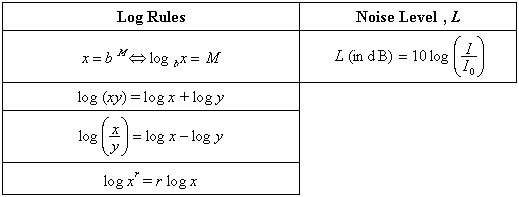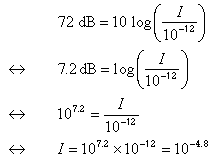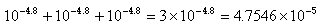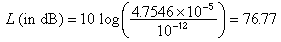# Occupational Health and Safety: Noise Levels and Decibels

## Question:

In a factory one machine produces a noise level of 72 dB. Two more similar machines each with a noise level of 72 dB are added in the same space. If, when machines are placed together, the sound intensities are additive (i.e. the combined intensity equals the sum of the intensities), what is the noise level in dB of the 3 machines?

## Solution

Basic rules for logarithms and exponents and the equation relating the noise level, L and the sound intensity, I.where

• Io = the reference sound intensity ( the minimum intensity detectable, 10-12 Watts/m2)
• dB is the unit for noise level = decibels

We can find the sound intensity of one machine as follows:With 3 identical machines, the sound intensity is:Now find the corresponding noise level in dBThe new noise level with 3 machines is 77 dB.Written by Louise Routledge, Tuesday, June 24, 1997